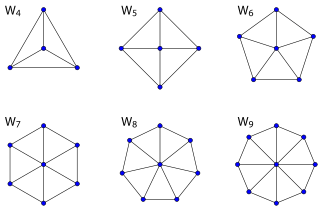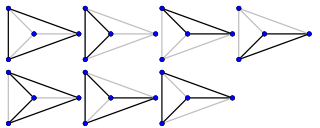# Wheel graph

Wheel graphSeveral examples of wheel graphs
Vertices n
Edges 2(n 1)
Diameter 2 if n>4
1 if n=4
Girth 3
Chromatic number 3 if n is odd
4 if n is even
SpectrumProperties Hamiltonian
Self-dual
Planar
Notation Wn

In the mathematical discipline of graph theory, a wheel graph is a graph formed by connecting a single vertex to all vertices of a cycle. A wheel graph with n vertices can also be defined as the 1-skeleton of an (n-1)-gonal pyramid. Some authors write Wn to denote a wheel graph with n vertices (n ≥ 4); other authors instead use Wn to denote a wheel graph with n+1 vertices (n ≥ 3), which is formed by connecting a single vertex to all vertices of a cycle of length n. In the rest of this article we use the former notation.

## Set-builder construction

Given a vertex set of {1,2,3,…,v}, the edge set of the wheel graph can be represented in set-builder notation by {{1,2},{1,3},…,{1,v},{2,3},{3,4},…,{v-1,v},{v,2}}.

## Properties

Wheel graphs are planar graphs, and as such have a unique planar embedding. More specifically, every wheel graph is a Halin graph. They are self-dual: the planar dual of any wheel graph is an isomorphic graph. Every maximal planar graph, other than K4 = W4, contains as a subgraph either W5 or W6.

There is always a Hamiltonian cycle in the wheel graph and there arecycles in Wn (sequence A002061 in the OEIS).The 7 cycles of the wheel graph W4.

For odd values of n, Wn is a perfect graph with chromatic number 3: the vertices of the cycle can be given two colors, and the center vertex given a third color. For even n, Wn has chromatic number 4, and (when n ≥ 6) is not perfect. W7 is the only wheel graph that is a unit distance graph in the Euclidean plane.

The chromatic polynomial of the wheel graph Wn is :In matroid theory, two particularly important special classes of matroids are the wheel matroids and the whirl matroids, both derived from wheel graphs. The k-wheel matroid is the graphic matroid of a wheel Wk+1, while the k-whirl matroid is derived from the k-wheel by considering the outer cycle of the wheel, as well as all of its spanning trees, to be independent.

The wheel W6 supplied a counterexample to a conjecture of Paul Erdős on Ramsey theory: he had conjectured that the complete graph has the smallest Ramsey number among all graphs with the same chromatic number, but Faudree and McKay (1993) showed W6 has Ramsey number 17 while the complete graph with the same chromatic number, K4, has Ramsey number 18. That is, for every 17-vertex graph G, either G or its complement contains W6 as a subgraph, while neither the 17-vertex Paley graph nor its complement contains a copy of K4.

1. Rosen, Kenneth H. (2011). Discrete Mathematics and Its Applications, 7th ed., McGraw-Hill, p. 655.
2. Trudeau, Richard J. (1993). Introduction to Graph Theory (Corrected, enlarged republication. ed.). New York: Dover Pub. p. 56. ISBN 978-0-486-67870-2. Retrieved 8 August 2012.
3. Buckley, Fred; Harary, Frank (1988), "On the euclidean dimension of a wheel", Graphs and Combinatorics, 4 (1): 23–30, doi:10.1007/BF01864150.
4. Faudree, Ralph J.; McKay, Brendan D. (1993), "A conjecture of Erdős and the Ramsey number r(W6)", J. Combinatorial Math. and Combinatorial Comput., 13: 23–31.